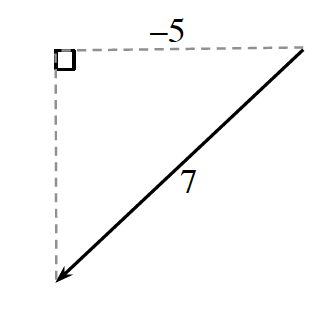### Home > PC3 > Chapter 6 > Lesson 6.2.4 > Problem6-129

6-129.

If $\cos(x)=-\frac{5}{7}$ and $π < x <\frac { 3 \pi } { 2 }$, what are the exact values of $\sin\left(x\right)$ and $\tan\left(x\right)$?